##### PostgreSQL AGE() Function With Examples

Calculating age is a very common task in our daily lives. For instance, in commercial applications, we frequently need to determine ages, such as people's age, years of service, etc. In Postgres, the AGE() function can be used to carry out these activities. It accepts two dates/timestamps and calculates the number of years, months, and days.

This post will teach us how to use the AGE() function in PostgreSQL.

How Does AGE() Function Work in PostgreSQL?

The AGE() function takes two timestamps as arguments, subtracts the second timestamp from the first one, and retrieves the resultant interval. The basic syntax of the AGE() function will look like this:

`AGE(First_TIMESTAMP, Second_TIMESTAMP);`

Example #1: How to Use the AGE() Function in Postgres?

In this example, we will pass two timestamps as arguments to the AGE() function:

`SELECT AGE('2018-12-12','2000-01-10');`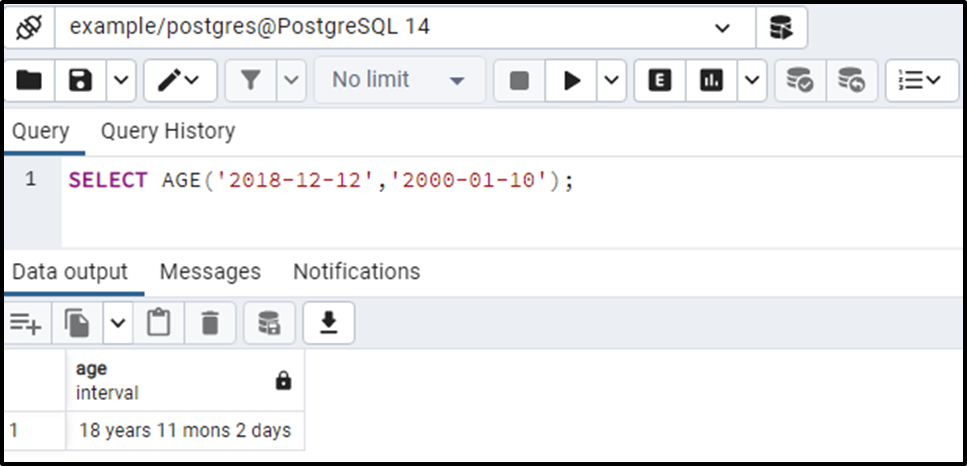The output verifies that the AGE() function returns the appropriate results.

Example #2: How to Use CURRENT_DATE Function With AGE() Function in Postgres?

Suppose we want to find the age of an employee whose birth date is “1990-01-10”. To do so, we can utilize the CURRENT_DATE() function with the AGE() function as follows:

`SELECT AGE(CURRENT_DATE, '1990-01-10');`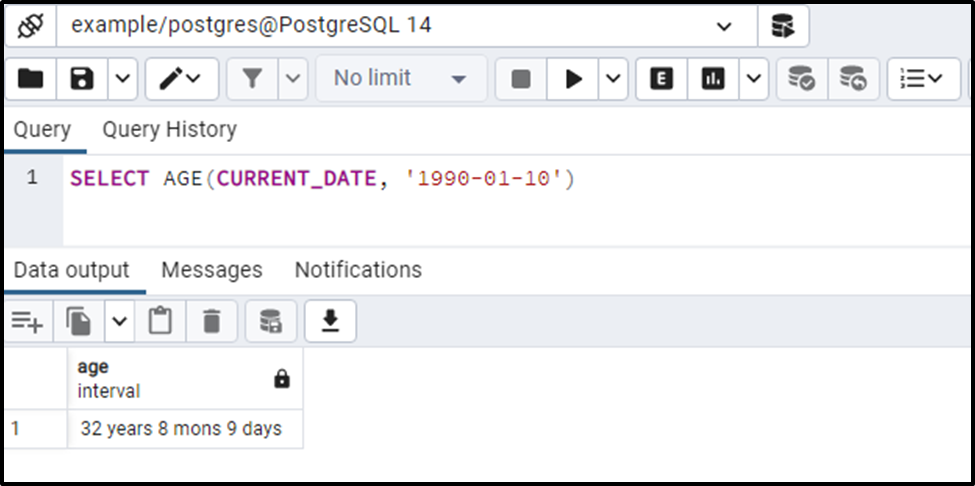The above snippet proves the working of the AGE() function, as it retrieves accurate results.

Example #3: How to Calculate the Age From the Current Date in Postgres?

You can find the age between the current date and the specified date by simply specifying the TIMESTAMP as follows:

`SELECT AGE(TIMESTAMP '2000-01-01');`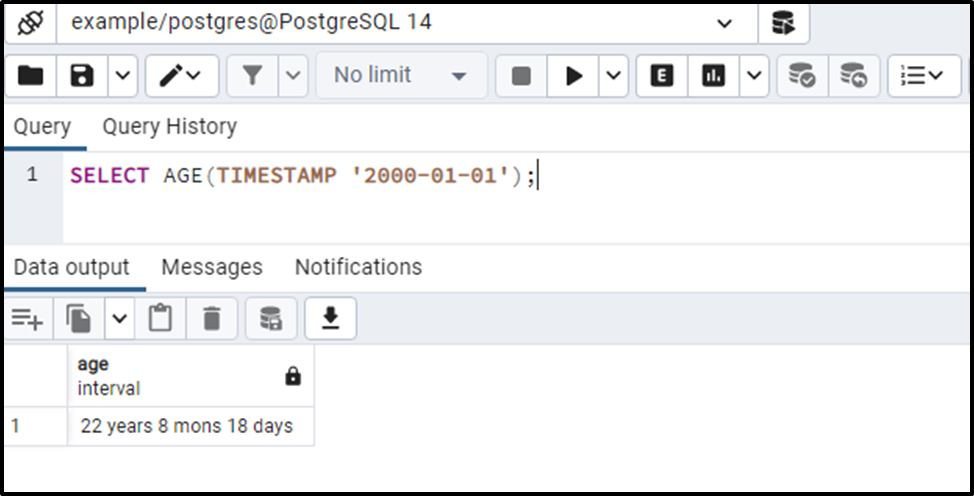This is how you can find the age between the current date and the specified date.

Example #4: How to Use AGE() Function on Table’s Data in Postgres?

We have created a table named student_bio that consists of the following records:

`SELECT * FROM student_bio;`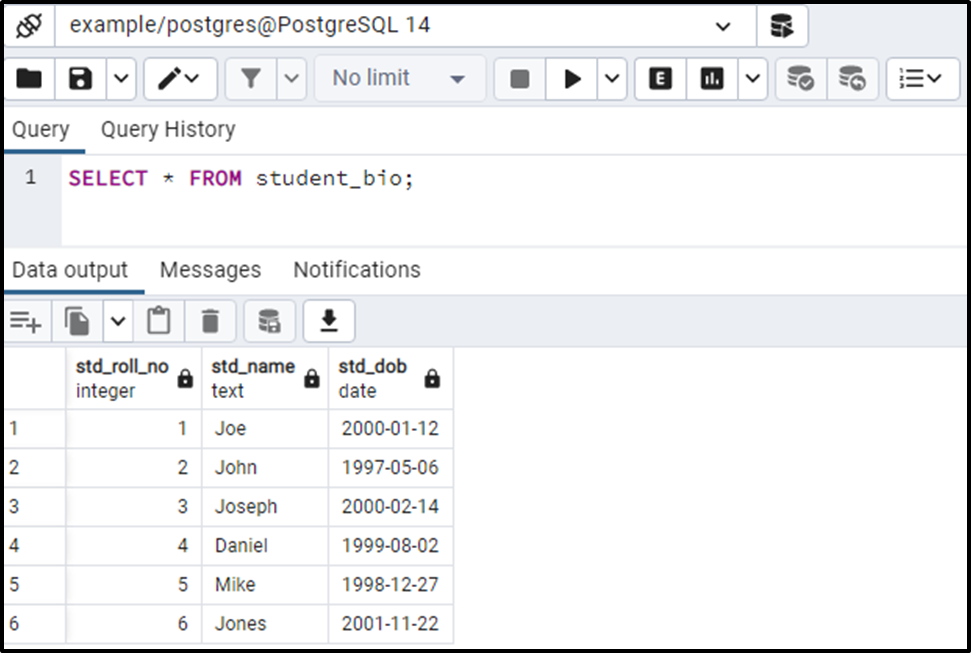Let’s say we have to find the Age of each student. To do so, we will use the AGE() function as follows:

```SELECT std_name, AGE(CURRENT_DATE, std_dob)
FROM student_bio;```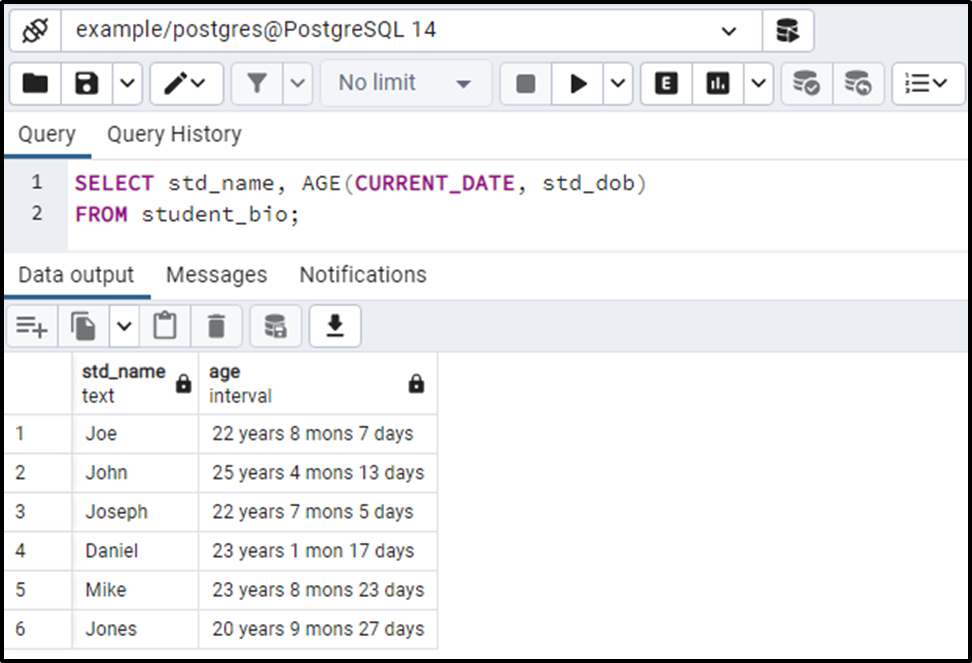The AGE() function successfully returned the age of each student.

Conclusion

In PostgreSQL, the AGE() function accepts two dates/timestamps and calculates the number of years, months, and days. The AGE() function takes two timestamps as arguments, subtracts the second timestamp from the first one, and retrieves the resultant interval. This write-up considered some practical examples to explain the working of the PostgreSQL AGE() function.